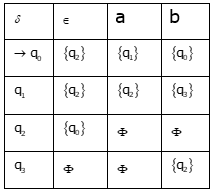# GATE Solved Paper 2017-19 - GATE 2017 Shift 2

>>>>>>>>GATE 2017 Shift 2

• A

I and IV only• B

II and III only• C

II, III and IV only• D

III and IV only• Option : D
• Explanation :
Is the language represented by regular expression
L(G) is the language generated by context free grammar
L(M) is the language accepted by Turing Machine

I. The problem a given regular expression R and a string w, is is a membership problem. Membership problem is decidable for Finite state machine and regular expression.

II. Given Context free grammar G, is L(G) is ϕ?, is emptiness problem for context free grammar. Emptiness problem is decidable for CFG by checking usefulness of start symbol.

III. A given context free grammar G, is L(G) is Σ* for some alphabet Σ?, is undecidable problem. We can’t check whether L(G) = Σ* or not but rather we can check complement of L(G) is ϕ. Since context free language are not closed under complement operation L(G) may be language accepted by Turing Machine and we can’t check emptiness for Turing machine.

IV. Given a Turing Machine M and a string w, is w Î L(M)?, is a membership problem for TM. Membership problem is not a decidable problem for TM.

• A

18• B

17• C

16• D

13• Option : A
• Explanation :
Number of blocks of main memory = 232 / 25 = 227
Number of blocks in cache memory = 512 = 29
1 Cache block points to 227 / 29 = 218 lines
So, Number of TAG bits required = 18 bits.

• A

Ø• B

{q0, q1, q2}• C

{q0, q1, q2}• D

{q0, q2, q3}• Option : C
• Explanation :
The given table for NFA-ϵ Transition isThe process is we start with ϵ-closure of q2 then for each input first take the transition then calculate ϵ-closure
q2 is the start for processing we take ϵ-closure which is {q0, q2 and process “aba”
q2 can reach q0, q1, and q2 after reading “aba”.

• A

I, II and IV only• B

II and III only• C

I and IV only• D

III and IV only• Option : D
• Explanation :
L1 is neither regular nor context free but context sensitive language.
L2 is context free, push any number of a’s and then for each b, pop two c’s until all b’s are over and this can be done by using only one stack.
L3 is not context free because we are not sure when to pop b and push a, because it is comparison between three consecutive terminals.
Clearly L3 is deterministic context free because we are sure of pushing a into stack first and on seeing b we are sure of popping a.
Statement III and IV are correct, option (D) is true.
Related Quiz.
GATE 2017 Shift 2